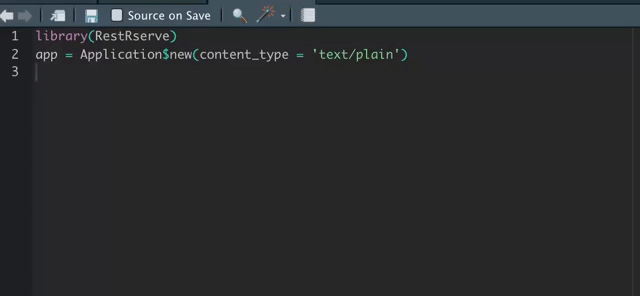# Quick Start Guide

## Introduction

Suppose you’ve developed a very useful algorithm or statistical model and you need to integrate it with some external system. Nowadays HTTP became de facto a lingua-franca for this kind of tasks.

In this article we will demonstrate how to use RestRserve to build a basic REST API.

## Workflow overview

Generally RestRserve workflow consists of several major steps:

## 2. Define logic

For simplicity we will use Fibonacci number calculation as an algorithm we want to expose.

calc_fib = function(n) {
if (n < 0L) stop("n should be >= 0")
if (n == 0L) return(0L)
if (n == 1L || n == 2L) return(1L)
x = rep(1L, n)

for (i in 3L:n) {
x[[i]] = x[[i - 1]] + x[[i - 2]]
}

return(x[[n]])
}

Create function which will handle requests.

fib_handler = function(.req, .res) {
n = as.integer(.req$parameters_query[["n"]]) if (length(n) == 0L || is.na(n)) { raise(HTTPError$bad_request())
}
.res$set_body(as.character(calc_fib(n))) .res$set_content_type("text/plain")
}

You may have noticed strange .req and .res argument names. Starting from RestRserve v0.4.0 these “reserved” names allows to benefit from autocomplete:Technically .req and .res are just empty instances of ?Request and ?Response classes exported by RestRserve in order to make autocomplete work.

app$add_get(path = "/fib", FUN = fib_handler) ## 3. Test endpoints Now we can test our application without starting it: request = Request$new(path = "/fib", parameters_query = list(n = 10))
response = app$process_request(request) cat("Response status:", response$status)
#> Response status: 200 OK
app$add_swagger_ui(path = "/doc", path_openapi = "/openapi.yaml", use_cdn = TRUE) ## 5. Start the app Now all is ready and we can start application with Rserve backend. It will block R session and start listening for incoming requests. backend = BackendRserve$new()
backend\$start(app, http_port = 8080)

## 6. Test it

Send request to calculate fibonacci number:

curl localhost:8080/fib?n=10

Check out a swagger UI in the browser: http://localhost:8080/doc